• 物联网大数据背景下城市交通现状分析及未来出行方式设想.pdf
•研究论文
•文档
• 从不同角度给出了模拟城市交通现状的方法和步骤.诸如乘客流网络、自行车网络、步行者网络流等的模拟方法;讨论了交通发生,交通分布、交通方式划分及交通分配模型的建立.并提出了解决城市交通问题的几种措施.
• 综合交通枢纽智能化系统设备现状分析及策略管理.pdf
• 国内车联网治理交通拥堵文献研究的现状分析与趋势思考——2011-2020年中国知网文献内容分析.pdf
•文档
• 杭州推行公共自行车交通系统现状与对策分析-论文.zip
• 黑龙江省交通科技发展环境建设现状及对策分析，彭晗，韩秀华，系统的阐述了交通科技发展环境的构成及其内涵，对黑龙江省交通科技发展环境建设的现状及存在的问题进行了详细的分析，提出了加强
• GPS导航系统在智能交通的应用现状及前景分析.pdf
• 我国自动驾驶发展现状及对综合交通体系的变革分析.pdf
• 交通出租车行业市场发展现状以与未来发展前景分析报告.doc文档
• 交通总务部的网站作进一步调查的政策和其他数据可能会感兴趣。 数据字典 这里每个数据集的所有功能 特征 类型 数据集 描述 年 整型 交通意外 事故发生年份 地区 目的 交通意外 事故发生地区 伤亡状况 目的 交通意外 ...
• 建筑施工中农民工安全状况调查分析，赵德刚，徐峰，本文采用调研的方法，在上海交通大学闵行校区11处建设工地进行了问卷调查和现场访谈，分析了农民工在宣传教育、治理监督、劳动防�
• 交通换乘功能是城市交通枢纽的核心功能之一，交通枢纽的交通换乘能力及枢纽内部客流交通组织状况是评价枢纽综合性能的重要指标。本文结合东直门交通枢纽工程实践就交通枢纽的交通换乘及客流交通组织评价问题进行探讨...
• 美国交通事故分析引言数据基本分析缺失值处理2.2 各州事故总数2.3时间处理3影响因素3.1天气状况3.2时间因素3.3路况因素4 预测分析5 总结 引言 该数据来源于kaggle网站中的一个项目，其原数据集（US_Accidents_Dec20...


美国交通事故分析
引言数据基本分析缺失值处理2.2 各州事故总数2.3时间处理
3影响因素3.1天气状况3.2时间因素3.3路况因素
4 预测分析5 总结

引言
该数据来源于kaggle网站中的一个项目，其原数据集（US_Accidents_Dec20）是一个数据量49列，共400多万数据量包含2016-2020年交通事故信息。 本文的目标是对这些数据进行统计分析，探寻发生事故最多的州，并探查什么时候容易发生事故，事故发生时天气状况，并对此做出可视化展示：总结分析所得信息，讲述美国发生事故的总体情况，找出影响事故发生以及影响事故严重程度的因子；最后对事故的严重程度进行预测分析。
数据基本分析
缺失值处理
查看原始数据的缺失值数量结果如下：
library('dplyr')
library('DMwR')
library('ggplot2')
library('lubridate')
#install.packages('Rmisc')
library(Rmisc)
library(mice)
#install.packages('VIM')
library(VIM)
library(zoo)
library(reshape2)
#导入数据
####----------------数据预处理-----------------------
#查看数据的变量名
colnames(data)
#查看数据的结构（4232541，49）
dim(data)
#查看数据中含有空值的列
colSums(is.na(data))
table(is.na(data))
#可以看到TMC含1516064；End_Lat含2716477；End_Lng含2716477
#Number含2687849；Temperature.F.89900；Wind_Chill.F.1896001；
#Humidity...95467；Pressure.in.76384；Visibility.mi.98668；
#列变量可视化展示
miscol = colSums(is.na(data))##列变量缺失值计数
miscol = sort(miscol,decreasing = T)  ##降序排列
miscol = miscol[miscol > 0]
p <- barplot(miscol,col = 'pink',axisnames=F,ylim=c(0,2000000),main = '列变量缺失值数量展示')
axis(side=1,p,labels = F)
labs <- names(miscol)首先，本文将变量值缺失个数多于总个数的20%的交通事故案例去除，此处共去除22913个案例；而后，因街道编号、风寒指数、风速、降水量的缺失值过多，所以将这几个变量移除；然后，移除与事故发生原因不相关变量；最后，采用均值插补的方法对温度、湿度、气压和能见度四个变量的缺失值进行插补，最终保留了27个变量。
#去除空值占比过大的行
r1 <- rowSums(is.na(data))/ncol(data) >= 0.2
#r1是缺失值大于0.2的行
data1 <- data[!r1, ]
rm(r1)
colSums(is.na(data1))
dim(data1)    #4209628
#将空值过多以及对研究影响因素作用不大的变量去除
data2 <- subset(data1, select = -c(ID,Source,TMC,End_Time,Zipcode,Timezone,
End_Lat,End_Lng,Number,
Distance.mi.,Description,Airport_Code,
Weather_Timestamp,Wind_Chill.F.,
Civil_Twilight,Nautical_Twilight,
Astronomical_Twilight))

#补齐缺失值
##用均值插补温度湿度气压能见度
fun1 <- function(x) {
x[is.na(x)] <- mean(x, na.rm = TRUE)
}
data2$Temperature.F. <- sapply(data2$Temperature.F., fun1)
data2$Humidity... <- sapply(data2$Humidity..., fun1)
data2$Pressure.in. <- sapply(data2$Pressure.in., fun1)
data2$Visibility.mi. <- sapply(data2$Visibility.mi., fun1)
##使用0填补降水量的缺失值
data2$Precipitation.in.[data1$Precipitation.in. %>% is.na()] <- 0
##测试各个变量之间有无显著线性关系
symnum(cor(data1 %>% select(Severity,Start_Lat,Start_Lng,Distance.mi.,Temperature.F.,Humidity...,Pressure.in.,
Pressure.in.,Visibility.mi.,Wind_Speed.mph.,Precipitation.in.), use="complete.obs"))
##对风速使用前值插补法
data2$Wind_Speed.mph.[1:5] <- zoo::na.locf(data1$Wind_Speed.mph.[1:5],fromLast = T)
data2$Wind_Speed.mph. <- zoo::na.locf(data1$Wind_Speed.mph.)
#table(is.na(data2$Precipitation.in.))  对27个变量的解释如下： “Severity” 显示事故的严重程度1-4 ； “Start_Time” 在本地时区事故的开始时间； “Street” 街道名称； “Side” 事故发生在哪一侧（L/R）； “City” 城市名； “County” 乡镇名； “State” 州名； “Country” 国家US ； “Temperature.F.” 温度； “Humidity…” 湿度； “Pressure.in.” 气压； “Visibility.mi.” 能见度； “Wind_Direction” 风向； “Weather_Condition” 天气状况（雨、雪等）； “Amenity” 附近有无便利设施； “Bump” 附近有无减速带； “Crossing” 附近有无交叉路口； “Give_Way” 附近有无该标志； “Junction” 附近是否是交界处； “No_Exit” 附近有无该标志； “Railway” 附近有无铁路； “Roundabout” 附近有无回旋处； “Station” 附近有无车站； “Stop” 附近有无停车标志； Traffic_Calming" 附近有无该标志； “Traffic_Signal” 附近有无该标志； “Turning_Loop” 附近有无转弯提示； "Sunrise_Sunset " 白天或晚上 2.2 各州事故总数 各州在2016-2020年间事故发生总数如下： #----------------分析各个州之间的数据---------- data3 <- data2 %>% group_by(State) %>% count %>% ungroup data3 %>% nrow data3在表中可以看到，事故发生量最多的州为CA（California），CA为美国第一大州，其经济总量在2019年约等于世界第五大经济体。经济繁荣必然伴随着交通的发达，同时交通事故发生的数量也会增大。 ##--------------------事故发生地图--------------- install.packages('maps') install.packages('ggmap') library(maps) library(ggmap) m <- borders("state",colour = "gray50",fill="white") df2 <- data2 %>% select(Start_Lat,Start_Lng) point <- df2[sample(nrow(df2),3000),] p1 <- ggplot(point,aes(x=Start_Lng,y=Start_Lat)) + m p1 + geom_point(color='steelblue',alpha=.6) + labs(title='美国交通事故地图')+ theme(plot.title = element_text(hjust = 0.5))2.3时间处理 原始数据包含了2016-2020年五年间的数据，五年事故总数如下表所示： 2016-2020事故总数 年份20162017201820192020数量4088687121868853279494001253847 由于事故数量庞大，本文提取出2020年的数据进行统计分析。 3影响因素 3.1天气状况 本文将数据中Weather_Condition变量提出进行分析，看到该变量有85个不同的值，于是本文选取总数大于5000 的11个值，对这11个天气状况与事故出现的严重程度的关系进行交叉分析如下图所示。 #---------------提取某一年的数据进行分析------- data2$year <- year(data2$Start_Time) summary(data2$year)
#选择2020年的数据
data4 <- data2[data2$year == 2020,] head(data4) colnames(data4) #--------------探究影响车祸程度的因子-------- data4$Severity <- as.factor(data4$Severity) data4$Wind_Direction <- as.factor(data4$Wind_Direction) data4$Weather_Condition <- as.factor(data4$Weather_Condition)#设为因子 str(data4) #查看数据类型 #封装绘图函数 # data：数据源，xlab：x轴数据，fillc：填充颜色， #pos：调整位置，xname：x轴标签文本，yname：y轴标签文本 fun_bar <- function(data, xlab, fillc, pos, xname, yname) { ggplot(data, aes(xlab, fill = fillc)) + geom_bar(position = pos) + labs(x = xname, y = yname) + coord_flip() + theme_minimal() } #-----------探索不同天气状况的时候对事故程度的影响------------- #天气状况很多，只画出一部分 rm(x1,x2,x3,x4) x1 <- data4 %>% group_by(Weather_Condition) %>% count %>% ungroup table(x1) #选取weather出现的数量大于5000的 #data_wea <- subset(data4, select = c(Weather_Condition,Severity)) #data_wea <- data_wea[data_wea$Weather_Condition != "",]
table(data4$Weather_Condition) #有空值 data5 <- data4[data4$Weather_Condition != "",]
df3 <- data5 %>% group_by(Weather_Condition) %>% dplyr::summarize(count=n())
#按照天气状况分类统计个数，挑选出个数大于5000的情况
df4 = df3[df3$count > 5000,] #选出11个 a <- df4$Weather_Condition #selected weathers
data6 <- data5[which(data5$Weather_Condition %in% a),] data7 <- subset(data6, select = c(Weather_Condition, Severity)) df5 <- data7 %>% group_by(Weather_Condition,Severity) %>% count df5 #交叉分析统计出11个最常出现的天气下对应程度1-4的个数 write.csv(df5,"D:/R数据/US_Accidents_2020_weather.csv", row.names=F) #ggplot(data7,aes(x=Weather_Condition,fill=Severity))+geom_bar(position="dodge") p3 <- fun_bar(data = data7, xlab = data7$Weather_Condition,
fillc = data7$Severity, #堆积条形图 pos = 'fill', xname = 'weather', yname = 'severity') p3 #rm(data7)从上图可以看出发生事故最多的11个天气状况都为云雾或雨天，其中暴雨（Heavy Rain）对事故的影响相对较大，更容易照成严重的交通事故。 随后，本文选择Temperature.F.变量进行分析，画出事故数量与温度变化的直方图： #-----------探索不同温度的时候对事故发生的影响------------- data8 <- subset(data4, select = c(Temperature.F.)) table(is.na(data8$Temperature.F.))   #有29615个缺失值
data8 <- na.omit(data8)   #删除缺失值
binsize <- diff(range(data8$Temperature.F.))/80 ggplot(data8, aes(x = Temperature.F.)) + geom_histogram(aes(y = ..density..), binwidth = binsize, fill = "pink", colour = "blue")+ stat_function(fun = dnorm, args = list(mean(data8$Temperature.F.), sd(data8$Temperature.F.)), size = 1) + geom_density(colour = "blue", size = 1) rm(data8)事故发生时的温度主要集中在50-70F左右，此温度接近人体最适宜温度，会有更多人的出门，增大事故发生的机率。 随后选择Humidity…、Pressure.in.、Visibility.mi.三个变量进行分析，画出三者分别对事故发生数量的影响如下： #-----------探索不同湿度的时候对事故发生的影响------------- data8 <- subset(data4, select = c(Humidity...)) table(is.na(data8$Humidity...))   #有32072个缺失值
data8 <- na.omit(data8)   #删除缺失值
binsize <- diff(range(data8$Humidity...))/40 ggplot(data8, aes(x = Humidity...)) + geom_histogram(aes(y = ..density..), binwidth = binsize, fill = "pink", colour = "blue")+ stat_function(fun = dnorm, args = list(mean(data8$Humidity...), sd(data8$Humidity...)), size = 1) + geom_density(colour = "blue", size = 1) rm(data8) #-----------探索不同气压的时候对事故发生的影响------------- data8 <- subset(data4, select = c(Pressure.in.)) table(is.na(data8$Pressure.in.))   #有32072个缺失值
data8 <- na.omit(data8)   #删除缺失值
binsize <- diff(range(data8$Pressure.in.))/40 ggplot(data8, aes(x = Pressure.in.)) + geom_histogram(aes(y = ..density..), binwidth = binsize, fill = "pink", colour = "blue")+ stat_function(fun = dnorm, args = list(mean(data8$Pressure.in.), sd(data8$Pressure.in.)), size = 1) + geom_density(colour = "blue", size = 1) rm(data8) #-----------探索不同能见度的时候对事故发生的影响------------- data8 <- subset(data4, select = c(Visibility.mi.)) table(is.na(data8$Visibility.mi.))   #有32072个缺失值
data8 <- na.omit(data8)   #删除缺失值
binsize <- diff(range(data8$Visibility.mi.))/40 ggplot(data8, aes(x = Visibility.mi.)) + geom_histogram(aes(y = ..density..), binwidth = binsize, fill = "pink", colour = "blue")+ stat_function(fun = dnorm, args = list(mean(data8$Visibility.mi.), sd(data8$Visibility.mi.)), size = 1) + geom_density(colour = "blue", size = 1) rm(data8)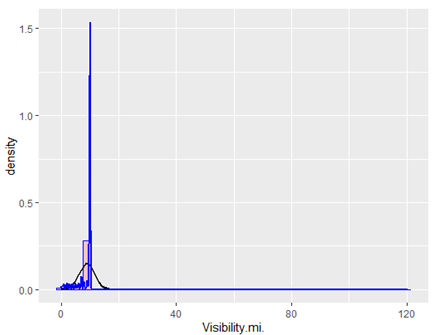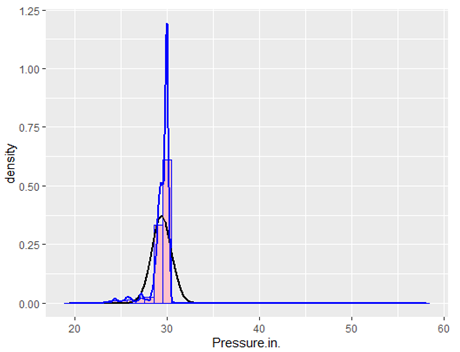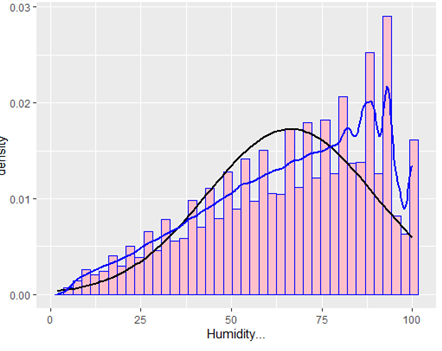在上图中可明显得看出，能见度低的时候正是事故的高发时期，能见度低会导致车辆和行人视野受限，没有足够的时间应对紧急情况，从而导致事故的发生。 美国大部分地区气候为地中海气候，全年温暖少雨，一年四季阳光充足，十分宜居。从上图也能看出，在天气状况较好时事故发生次数最多。很大原因是天气晴朗时，会有更多人走出家门游玩，人流量和车流量都会相应增大，发生交通事故的可能性也会上升。相比之下，天气状况不好时事故发生数量反而降低，这种天气下，人们会减少出行次数。 3.2时间因素 首先，对每天中交通事故发生时间进行分析，作出折线图： #---------------分析各个时间段发生事故的数据---------- head(data2) data2$Start_Time <- as.POSIXct(data2$Start_Time)#设置时间格式 data2$St_hour <- hour(data2$Start_Time) df1 <- data2 %>% group_by(St_hour) %>% dplyr::summarize(count=n()) p2 <- ggplot(df1,aes(x=St_hour,y=count,fill='pink')) p2 + geom_line(linetype=1,color='blue')+ geom_point(size = 3, shape = 21, colour = "blue",fill='white')+ geom_area(fill = 'pink',alpha=.7)+ scale_x_continuous(breaks = c(0:23),name='hour')+ labs(title='各时间车祸发生次数')+ theme(plot.title = element_text(hjust = 0.5)) #rm(df1) data2$mon <- month(data2$Start_Time) df1 <- data2 %>% group_by(mon) %>% dplyr::summarize(count=n()) p2 <- ggplot(df1,aes(x=mon,y=count,fill='pink')) p2 + geom_line(linetype=1,color='blue')+ geom_point(size = 3, shape = 21, colour = "blue",fill='white')+ geom_area(fill = 'pink',alpha=.7)+ scale_x_continuous(breaks = c(0:23),name='month')+ labs(title='各月车祸发生次数')+ theme(plot.title = element_text(hjust = 0.5)) write.csv(data2,"D:/R数据/data2.csv", row.names=F)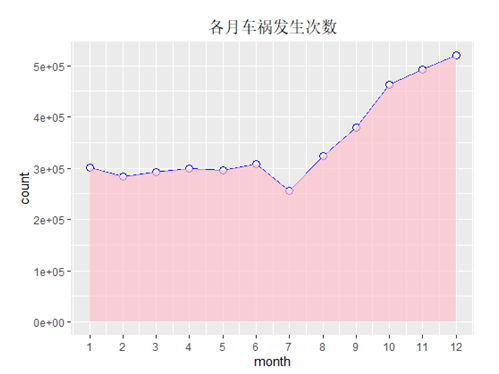从上图可知，一天之中两个事故发生高峰期位于早上6-9点与下午4-6点之间，这与人们的生活作息相关，如这两段时间内人们会集中上下班和上学放学。 对于每年中交通事故在各月中出现的次数进行分析，探查其季节规律，绘出折线图如下：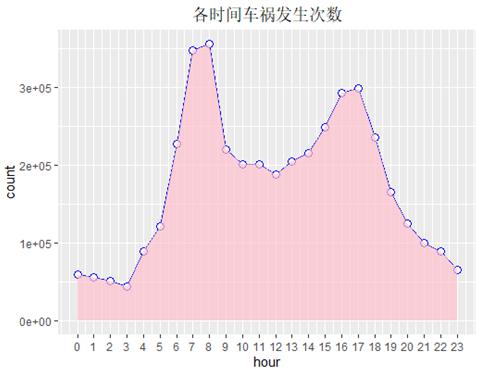由上图可知，2016-2020年间美国在上半年的事故数明显少于后半年的事故数。 3.3路况因素 对于路况、白天黑夜这样的环境因素对于事故发生的影响，我们选取多个逻辑变量进行探寻，绘制出环境因素的堆积条形图如下： #------------探索定性变量对事故的影响-------------- library(plyr) library(magrittr) data9 <- subset(data4, select = c(Crossing, Sunrise_Sunset, Precipitation.in., Traffic_Signal, Give_Way, Junction, Bump, Station, Railway, Traffic_Calming, Turning_Loop)) #Traffic_condition <- data1 %>% select(Crossing,Sunrise_Sunset,Precipitation.in.,Traffic_Signal,Give_Way,Bump) #将降雨量转化为逻辑变量 data9$Precipitation.in.[data9$Precipitation.in. > 0] <- 'True' data9$Precipitation.in.[data9$Precipitation.in. == 0] <- 'False' head(data9) #查看各个变量的结构 table(data9$Sunrise_Sunset) #Sunrise_Sunset中有空值，删去
data9 <- data9[data9$Sunrise_Sunset != "",] data9$Sunrise_Sunset[data9$Sunrise_Sunset == 'Day'] <- 'True' data9$Sunrise_Sunset[data9$Sunrise_Sunset == 'Night'] <- 'False' df6 <- rbind(colSums(data9=='True'),colSums(data9=='False')) %>%cbind(Boolean=c('True','False')) %>% data.frame() data_env <- melt(df6, id.vars = 'Boolean', variable.name = 'Environ', value.name = 'Accidents' ) str(data_env) #Accidents为字符型数据，转换为数值型 data_env$Accidents <- as.numeric(data_env$Accidents) x1 <- ddply(data_env,'Environ',transform, percent_num = Accidents/sum(Accidents)*100) p4 <- ggplot(x1,aes(x=Environ,y=percent_num,fill=Boolean)) p4 + geom_bar(stat = 'identity',color='blue')+ scale_fill_brewer(palette = 11)+ geom_text(aes(label=paste(round(percent_num),"%"),vjust=-0.3))+ labs(title='环境因素堆积条形图')+ theme(plot.title = element_text(hjust = 0.5))从上图可知，交通事故主要发生道路交界处、交通信号灯、车站等地方。这些地方往往路况比较复杂，车流量和人流量都比较大，容易发生交通事故。尤其是十字交叉路口，四个方向的车汇集此处，相比普通路段，碰撞的概率大大增加。 4 预测分析 本文选取Crossing、Sunrise_Sunset、Precipitation.in.、Traffic_Signal、Junction、Station、Railway、Temperature.F.、Humidity…、Pressure.in.、Visibility.mi.、St_hour、mon这13个因子对事故的严重程度建立模型进行预测。其中，将逻辑变量转化为数值型的0、1，将所有因子转化为数值型变量进行xgboost模型的建立。 #--------------------预测-------------------- install.packages('xgboost') library(xgboost) #由上述分析中，将有用指标提取出来 data4$mon <- month(data4$Start_Time) data0 <- subset(data4, select = c(Crossing, Sunrise_Sunset, Precipitation.in., Traffic_Signal, Junction, Station, Railway, Temperature.F., Humidity..., Pressure.in., Visibility.mi., St_hour, mon, Severity)) table(data0$Sunrise_Sunset)
data0 <- data0[which(data0$Sunrise_Sunset!=''),] data0$Sunrise_Sunset[data0$Sunrise_Sunset=='Night'] <- 'False' data0$Sunrise_Sunset[data0$Sunrise_Sunset=='Day'] <- 'True' data0[data0=='False'] <- 0 data0[data0=='True'] <- 1 str(data0) colnames(data0) #将变量值转化为数值型 data0[,1:13] <- data0[,1:13] %>% apply(2,as.numeric) %>% data.frame() y <- data0$Severity
X <- scale(data0[,-14],scale = T) %>% data.matrix()
bst <- xgb.cv(data = X,label = y,max.depth=12,eta=0.007,nrounds = 10,
objective = "multi:softmax",num_class=5,nfold = 5,booster='gbtree')

xgmodel <- xgboost(data = X,label = y,max.depth=12,eta=0.007,nrounds = 10,
objective = "multi:softmax",num_class=5,booster='gbtree')
pre_y <- predict(xgmodel,X)#预测值
accuracy <- sum(pre_y==y)/length(y)#计算准确率
accuracy


最后计算出模型的准确率为0.816472，模型比较合适。
5 总结
美国交通事故发生最多的州是加利福尼亚州，并且交通事故数量逐年上升。影响交通事故的主要因素有天气、路况、出行时间等。天气越好，路况越差，事故发生越频繁；出行时间则与人们的生活作息有关。各城市经济越发达，事故发生次数也越多。我们在外出之时，需要注意路况，在交叉路口、转弯标识等处注意车辆和行人。
展开全文• 郑州市交通拥堵分析及对策研究，代小瑞，余丰茹，随着城市化进程加快和机动车保有量的飞速增长，郑州市交通拥堵已成为当前城市发展过程中亟待解决的问题，从交通与城市土地利用协
• 浅析城市交通现状及问题 近几年来，随着国民经济的快速增长，城市化进程不断加快，城市人口也在不断集中。拥有数百万甚至上千万人口的大城市的数量不断增加。城市化进程带动了交通需求的快速增长。与此同时，大城市...
浅析城市交通现状及问题 电子科技大学格拉斯哥学院 2017级 陈玉龙
近几年来，随着国民经济的快速增长，城市化进程不断加快，城市人口也在不断集中。拥有数百万甚至上千万人口的大城市的数量不断增加。城市化进程带动了交通需求的快速增长。与此同时，大城市的机动车数量正在不断增加，也促进了交通需求的增加。交通需求的快速增长使得城市道路负荷加重，交通堵塞，行车混乱等现象屡屡发生。其中，交通拥堵问题尤其严重，已经阻碍到城市经济的发展，也成为人们日常讨论的话题之一。
目前，交通拥堵问题主要有以下几个因素构成：
城市规模的扩大和交通设施的落后 随着城市建设的发展和扩大，市中心城区的地位日益突出，流动人口数不断增加。人流量的增加带来的巨大压力使得城区道路无法承受。城市道路网的建设速度远不及交通量的增长速度。其次，城市道路建设的增加主要在于新城区及周边延伸的区域，旧城区的道路网依然落后，难以满足市民的出行需求。旧城区的道路规划还停留在几十年前，并不适应现今的交通状况。因此，交通拥堵现象往往发生于中心旧城区，而新城区很少发生交通堵塞现象。旧城区的道路狭窄，道路网密度较高，交叉口繁多，造成许多出行的不便。城市道路的不足和机动车数量的快速增长 随着市民生活水平的提高，家庭拥有小轿车的数量逐渐上升。轿车已经成为工薪阶层家庭常用的代步工具。汽车购买量增长加快，但城市的道路建设却无法跟上。机动车数量正以15%的速度增长，而道路建设的增长率仅为3%。这种增长的极度不平衡导致道路交通压力不断加大，交通拥堵的频率越来越高。同时，许多城市内部道路并未扩宽，道路狭窄，基础设施落后，不仅造成不便而且极易引发交通拥堵现象。市民守法意识的薄弱 许多城市居民在出行时十分随意，不论是行人还是驾驶员，都频繁做出违反交通法规的行为。近年来随着更加严格的交通法规的制定和执行，违法行为次数减少，但仍不少人尝试利用交通法律的漏洞和监管不力来“方便”自己。非机动车便是其中一个典型的例子，由于城市出行距离不长，加上堵车现象的频发，不少市民选择摩托车电动车等非机动车辆出行，而这些车辆所受限制较少，处罚制度不完善，使得电动车随意变道，乱穿马路，无视信号灯的行为不断发生。这种行为使得交通拥堵现象更易发生，同时还留下了很多安全隐患。
针对以上问题，若想减少交通拥堵状况的发生，就要采取有效的对策。在许多缓解交通压力的方法中，我认为有如下几点较为关键：
加快道路建设 主城区是交通拥堵频发地段，若想从根本上解决问题，就要完善道路的建设，重新规划道路网。将一些道路扩宽，增加车道数，重新设计道路网，将一些地方改为单行道，能极大缓解主城区的交通压力。同时还应完善道路基础设施，信号灯、指示牌必不可少，非机动车道和机动车道的界限也要十分明确，防止交通混乱的现象发生，同时也使市民出行更加安全。限制机动车的增长 由于市民生活水平的提高，机动车的购买量增长十分迅速，远高于道路建设速度。限制机动车的购买，可以从一定程度上缓解道路供需不平衡的情况。同时，近年来推出的共享单车产品改变了部分市民的出行方式，也减少了机动车的流量，缓解了交通压力。因此，有关部门应出台措施限制机动车的购买，同时鼓励共享单车出行，既能缓解交通压力，又能保护环境。增强市民守法出行的意识 交通规则的修订使得城市出行变得比以往更加有序，然而仍不能杜绝乱象的发生。对非机动车的管理需要变得更加严格，采取明确的处罚措施，减少非机动车乱穿马路，胡乱变道的情况。同时宣传文明出行，增强市民的守法意识，减少行人乱穿马路，结伴闯红灯的现象，使得出行更加井然有序，减少交通拥堵的几率。
若要阻止交通拥堵的情况发生，就必须采取多方面措施并落实执行，从法律政策，宣传教育，道路建设等方面多管齐下，提供给市民一个安全出行环境，使得出行更加高效快捷。
展开全文• 对山区双车道旅游公路的交通特性进行了较为...速度数据的分析结果表明:山区双车道旅游公路 的现状车速偏高;陡坡路段速度离散性较大。这不仅与货车比例等交通组成有关,而且与陡坡路段自身的道路特 性也有较大的相关性。
• 描述城市交通现状、呈现演变规律、预测未来发展趋势，并专注拥堵成因及解决对策的研究。本年报由高德地图联合“中国社会科学 院社会学研究所”、“未来交通与城市计算联合实验室”、“阿里云”、“重庆交通大学蔡...jt
• 针对航空器服务架次不能精确刻画空中交通复杂程度的现状,提出一种通过多指标度量空中交通复杂性的方法。首先通过实测雷达数据计算得出9个空中交通复杂性评价指标值,并对复杂性指标进行了相关性分析及因子分析的适用...
• 根据南昌市2000年至2003年的道路交通安全现状，利用统计分析方法，对道路交通事故的成因、时空分布、事故形态等进行了分析和研究，并针对南昌市的交通事故的特点与规律，提出了预防交通事故的若干措施。
• 分析中国农村公路交通安全现状及趋势的基础上，从人、车、路和环境4个方面分析农村公路交通安全的影响因素并提出对策。分析表明：人的因素主要表现为驾驶技术不过关、安全意识淡薄；车的因素主要表现为机动车车型...
• 基于对城市交通现状分析，提出了多车道交通流控制模型，并对此进行了研究与稳定性分析...